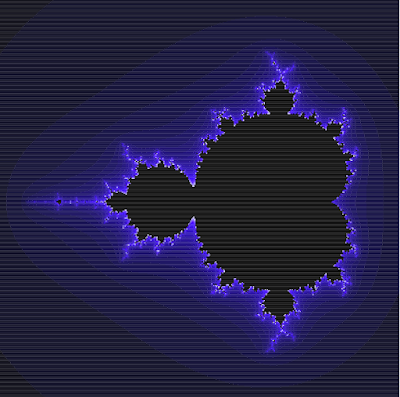## Tuesday 11 June 2019

### Mandelbrot Set in SQL using SVG with RLE

A popular SQL party trick is to generate the Mandelbrot set using Common Table Expressions (CTEs) to implement the required iteration.   The usual demo outputs the image using ASCII art:

This is impressive in its own way...  but really it's like, so 70's, man.

Here in the 21st century we have better tooling for describing graphics using text - namely, Scalable Vector Graphics (SVG).  And best of all, it's built right into modern browsers (finally!).  So here's the SQL Mandelbrot set brought up to date with SVG output.

A straightforward conversion of the quert is relatively easy.  Simply render each cell pixel as an SVG rect element of size 1, and use a grayscale colour scheme. But  a couple of improvements produce a much better result:
1. A more varied colour palette produces a nicer image
2. Using one SVG element per cell result in a very large file, which is slow to render.  There's a lot of repeated pixels in the raster, so a more compact representation is possible
The colour palette is easily improved with a bit of math (modulo cumbersome SQL syntax).  Here I use a two-ramp palette, sweeping through shades from black to blue, and then through tints to white.

A simple way of reducing raster size is to use Run-Length Encoding (RLE).  This works well with SVG because the rect element can simply be extended by increasing the width attribute.  The tricky part is using SQL to merge the rows for contiguous same-value cells .  As is often the case, a straightforward procedural algorithm requires some cleverness to accomplish in SQL.  It had me stumped for a while.  The solution seemed bound to involve window functions, but trying various combinations of the multitudinous options available didn't produce the desired result.  Then I realized that the problem is isomorphic to that of merging contiguous date ranges.  That is a high-value SQL use case, and there's numerous solutions available.  The two that stand out are (as discussed here):
• Start-of-Group - this approach uses a LAG function to flag where the group value changes, followed by a running SUM to compute a unique index value for each group (run, in this case).  Group rows are then aggregated on the index
• Tabibitosan - this is a clever and efficient approach, but is harder to understand and less general
The solution presented uses Start-of-Group, for clarity.  RLE reduces the number of SVG elements to about 12,000 from 160,000, and file size to 1 MB from 11 MB, and hence much faster loading and render time in a web browser.

Here's the output image, with the SQL query producing it below (also available here).Here's how the query works:
1. x is a recursive query producing a sequence of integers from 0 to 400 using standard SQL
2. z is a recursive query creating the Mandelbrot set on a 400x400 grid.  A scale and offset maps the grid cell ordinates into the complex plane, centred on the Mandelbrot set.  The query computes successive values of the set equation for each cell.  A cell is terminated when it is determined that the equation limit is unbounded.
3. itermax selects the maximum iterations for each cell.  This result set contains the final result of the Mandelbrot computation
4. runstart finds and flags the start of each RLE "run" group for each row of the raster
5. runid computes an id for each run in each row
6. rungroup groups all the cells in each run and finds the start and end X index
7. plot assigns a colour to each run, based on the iteration limit i
8. the final SELECT outputs the SVG document, with rect elements for each run

```WITH RECURSIVE
x(i) AS (
VALUES(0)
UNION ALL
SELECT i + 1 FROM x WHERE i ≤ 400
),
z(ix, iy, cx, cy, x, y, i) AS (
SELECT ix, iy, x::FLOAT, y::FLOAT, x::FLOAT, y::FLOAT, 0
FROM
(SELECT -2.2 + 0.0074 * i, i FROM x) AS xgen(x, ix)
CROSS JOIN
(SELECT -1.5 + 0.0074 * i, i FROM x) AS ygen(y, iy)
UNION ALL
SELECT ix, iy, cx, cy, ```
```      x*x - y*y + cx AS x, y*x*2 + cy, i + 1
FROM z
WHERE x*x + y*y < 16.0
AND i < 27
),
itermax (ix, iy, i) AS (
SELECT ix, iy, MAX(i) AS i
FROM z
GROUP BY iy, ix
),
runstart AS (
SELECT iy, ix, I,
CASE WHEN I = LAG(I) OVER (PARTITION BY iy ORDER By ix)
THEN 0 ELSE 1 END AS runstart
FROM itermax
),
runid AS (
SELECT iy, ix, I,
SUM(runstart) OVER (PARTITION BY iy ORDER By ix) AS run
FROM runstart
),
rungroup AS (
SELECT iy, MIN(ix) ix, MAX(ix) ixend, MIN(i) i
FROM runid
GROUP BY iy, run
),
plot(iy, ix, ixend, i, b, g) AS (
SELECT iy, ix, ixend, i,
CASE
WHEN i < 18 THEN (255 * i / 18.0 )::integer
WHEN i < 27 THEN 255
ELSE 0 END AS b,
CASE
WHEN i < 18 THEN 0
WHEN i < 27 THEN (255 * (i - 18) / (27 - 18 ))::integer
ELSE 0 END AS g
FROM rungroup
ORDER BY iy, ix
)
SELECT '<svg viewBox="0 0 400 400" '```
`  || ' style="stroke-width:0" xmlns="http://www.w3.org/2000/svg">' `
```  || E'\n'
|| string_agg(
'<rect style="fill:rgb(' ```
```      || g || ',' || g || ',' || b || ');"  '
|| ' x="' || ix || '" y="' || iy
|| '" width="' || ixend-ix+1 || '" height="1" />', E'\n' )
|| E'\n' || '</svg>' || E'\n' AS svg
FROM plot;
```